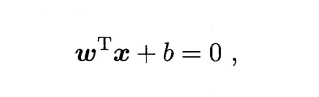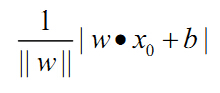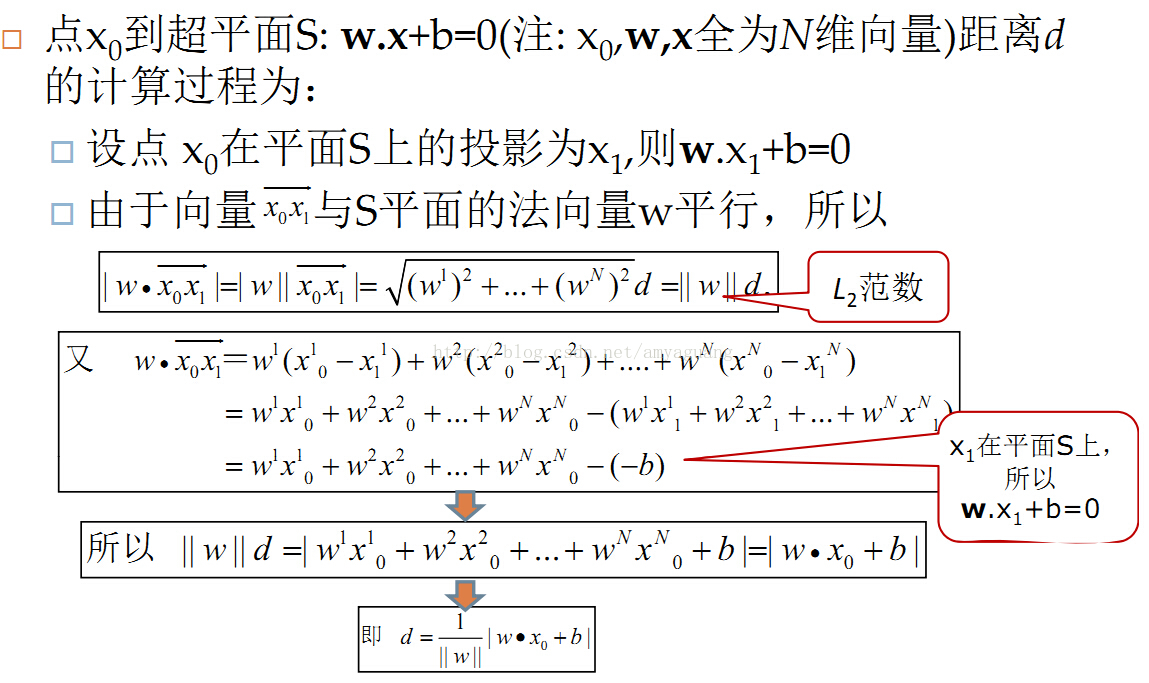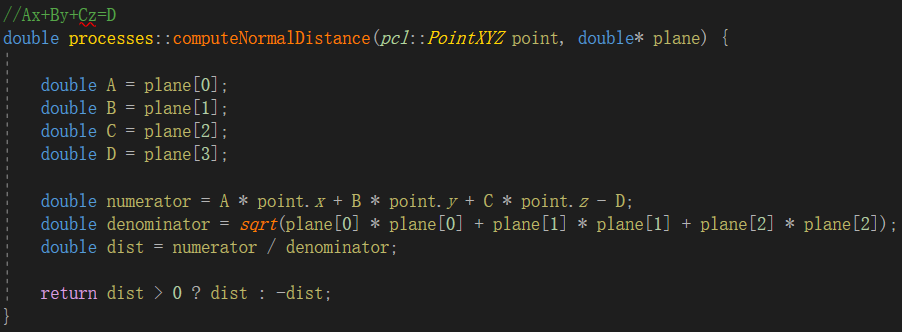• 支持向量机就是找到划分超平面中...其中任意点到超平面距离公式y为： 上述是点在超平面的正一侧的情形，如何点再在超平面负的一侧，只需在公式前加-号即可。 综上所述，点与超平面距离公式可表示为： yi*y ...
支持向量机就是找到划分超平面中间隔最大的超平面，设定划分超平面的线性方程为：其中 w 决定了超平面的方向 ; b 为位移项，决定了超平面与原点之间的距离.显然，划分超平面可被法向量 ω 和位移 b 确定 。

其中任意点到超平面的距离公式y为：上述是点在超平面的正一侧的情形，如何点再在超平面负的一侧，只需在公式前加-号即可。

综上所述，点与超平面的距离公式可表示为：
yi*y

展开全文• 文章目录1、超平面一般表示形式2、超平面的法向量3、点到超平面的距离4、平行超平面之间的距离公式   1、超平面一般表示形式 在n维空间中，设任意点坐标为 xT=[x(1),x(2),...x(n)]T∈Rnx^T=[x^{(1)},x^{(2)},...x^...
文章目录1、超平面一般表示形式2、超平面的法向量3、点到超平面的距离4、平行超平面之间的距离公式

1、超平面一般表示形式
在n维空间中，设任意点坐标为
$x=[x^{(1)},x^{(2)},...x^{(n)}]^T\in{R^n}$
设超平面参数
$w=[w^{(1)},w^{(2)},...w^{(n)}]^T\in{R^n}$
$b\in{R}$
则超平面方程可表示为
$w^T x+b=0\tag{1}$

2、超平面的法向量
超平面的法向量满足：超平面中任意向量都与该法向量垂直。设超平面上的两个点为$x_1$和$x_2$，分别满足：
$w^T x_1+b=0\tag{2}$
$w^T x_2+b=0\tag{3}$
两式相减，可得
$w^T (x_1-x_2)=0\tag{4}$
记 $\bm{v}=(x_1-x_2)$，由于$x_1$和$x_2$是任取的，故 $\bm{v}$ 表示超平面上的任意向量。这时我们可以发现，式$(4)$的含义恰好是：平面上任意一个向量都与 $w$ 相互垂直，因此 $w$ 就是超平面$w^T x+b=0$的一个法向量。

3、点到超平面的距离
记超平面外一点为 $x_0$ ，记点 $x_3$ 在超平面$w^T\cdot x+b=0$上的投影点为 $x_0'$，满足：
$w^T\cdot x_0'+b=0\tag{5}$
则有向量 $\bm{u}=(x_0-x_0')$ 与平面$w^T x+b=0$的法向量$\bm{w}$互相平行，则两者的数量积：
$w^T(x_0-x_0')=w\cdot (x_0-x_0')=|w|*|x_0-x_0'|*cos(0~or~\pi)=\pm|w|*d\tag{6}$
其中 $d=|x_0-x_0'|$ 即为待求的点到超平面间的距离。
另一方面，根据式$(5)$消去可得
$w^T(x_0-x_0')=w^Tx_0-w^Tx_0'=w^Tx_0-(-b)=w^Tx_0+b\tag{7}$
结合$(6)(7)$，考虑到 $d\ge0$，可得
$d=\frac{|w^Tx_0+b|}{|w|}\tag{8}$
这里上式中的 $|w|$ 表示 $w$ 的模长，模长作为绝对值概念的推广，在欧式空间中，模长常常称为L2范数（也称为Euclidean范数或者Frobenius范数）：
$||w||_F=\sqrt{(w^{(1)})^2+(w^{(2)})^2+...+(w^{(n)})^2}$
所以，$d$ 的表达式即为：
$d=\frac{|w^Tx_0+b|}{||w||_F}\tag{9}$
这样来看，平面直角坐标系下的点到直线距离公式便是上式的一个特例。

4、平行超平面之间的距离公式
趁热打铁，继续推导平行超平面间的距离公式，设两个不重合的平行超平面分别为：
$w_1^T x+b_1=0$
$w_2^T x+b_2=0$
由于两个超平面互相平行，因此由 $2$ 中对法向量的讨论可知，两个超平面的法向量互相平行，我们取两个互相重合的法向量，即
$w=w_1=w_2$
则可得
$w^T x+b_1=0\tag{10}$
$w^T x+b_2=0\tag{11}$
设 $P(x_0)$ 为平面1上的一个点，即满足：
$w^Tx_0+b_1=0\tag{12}$
则根据点到超平面的距离公式可得点 $P(x_0)$ 到超平面2的距离 $d$ 满足：
$d=\frac{|w^Tx_0+b_2|}{||x_0||_F}=\frac{|-b_1+b_2|}{||w||_F}$
上式最后一步用到了式$(12)$。最后我们得到了平行超平面之间的距离公式为
$d=\frac{|b_2-b_1|}{||w||_F}\tag{13}$


展开全文线性代数 矩阵 动态规划
  点到平面距离公式推导如下图所示，详细信息参见百度百科：https://baike.baidu.com/item/%E7%82%B9%E5%88%B0%E5%B9%B3%E9%9D%A2%E8%B7%9D%E7%A6%BB/10690055?fr=aladdin该计算方法在C++中的实现代码如下：展开全文算法 c++
• 点到平面距离公式 准备知识 平面的一般式方程 Ax +By +Cz + D = 0 其中n = (A, B, C)是平面的法向量，D是将平面平移到坐标原点所需距离（所以D=0时，平面过原点） 向量的模（长度） 给定一个向量V（x, y, z)...
点到平面的距离公式

准备知识

平面的一般式方程

Ax +By +Cz + D = 0

其中n = (A, B, C)是平面的法向量，D是将平面平移到坐标原点所需距离（所以D=0时，平面过原点）

向量的模（长度）

给定一个向量V（x, y, z),则|V| = sqrt(x * x + y * y + z * z)

向量的点积（内积）

给定两个向量V1(x1, y1, z1)和V2(x2, y2, z2)则他们的内积是

V1V2 = x1x2 + y1y2 + z1z2

点到平面的距离

有了上面的准备知识，则求点到直线的距离不再是难事，有图有真相如果法相量是单位向量的话，那么分母为1

转载自：http://www.cnblogs.com/graphics/archive/2010/07/10/1774809.html

展开全文推导 公式
• ## SVM：任意点到超平面的距离公式

万次阅读 多人点赞 2018-10-18 11:05:06
任意点 到超平面距离公式 在样本空间中，划分超平面可通过如下线性方程来描述: 其中 w 决定了超平面的方向 ; b 为位移项，决定了超平面与原点之间的距离.显然，划分超平面可被法向量 ω 和位移 b 确定 。 ...
• 其中n = (A, B, C)是平面的法向量，D是将平面平移到坐标原点所需距离（所以D=0时，平面过原点） 向量的模（长度） 给定一个向量V（x, y, z),则|V| = sqrt(x * x + y * y + z * z) 向量的点积（内积） 给定两个...
• 预备知识： (1) 平面的一般表达式： 其中，n=(A,B,C)是平面的法向量，D决定了平面与原点之间的距离，当D=0时，平面经过原点。...点到直线的距离公式推导：   参考博客：http://www.cnblogs.co...
• SVM算法基础之点到平面距离公式推导 SVM算法基础之点到平面距离公式推导 平面的一般式方程 Ax +By +Cz + D = 0 其中n = (A, B, C)是平面的法向量，D是将平面平移到坐标原点所需距离（所以D=0时，平面过原点） ...机器学习
• 我的机器学习教程 「美团」算法工程师带你入门机器学习  以及  「三分钟系列」数据结构与算法  已经开始更新了，欢迎大家订阅...在感知机模型中，输入空间中任意一点 到超平面S的距离： 其推导过程如下：机器学习 统计学习 几何
• 空间直线方程及两直线的夹角 空间直线的一般方程 方向向量 直线的点向式（对称式）方程 两直线的夹角 两直线相互垂直和平行的充分必要条件
• 其中n = (A, B, C)是平面的法向量，D是将平面平移到坐标原点所需距离（所以D=0时，平面过原点） 向量的模（长度） 给定一个向量V（x, y, z),则|V| = sqrt(x * x + y * y + z * z) 向量的点积（内积） 给定两个...
• 在感知机模型中，输入空间中任意一点 到超平面S的距离：其推导过程如下：
• 在这里对上面的公式推导做个解释，由于2个向量的点积的公式为，而向量想x0x1又与超平面平行，所以2者形成的夹角0或者180，也就是cosα=+1或者-1，所以2个向量点积的绝对值为2者模的乘积。也就是L2范数那一行成立的...机器学习
• 关于支持向量机（SVM）I型中，对任意x0x_0x0​来说，为何x0x_0x0​到超平面ωTX+b=0\omega^TX+b=0ωTX+b=0的距离公式中，必满足以下式子：∣ωTx0+b∣=1|\omega^Tx_0+b|=1∣ωTx0​+b∣=1，？？？ 其实可以这样看： ...svm
• 已知三点p1（x1,y1,z1），p2(x2,y2,z2)，p3...为此做向量p1p2（x2-x1,y2-y1,z2-z1), p1p3(x3-x1,y3-y1,z3-z1),平面法线和这两个向量垂直，因此法向量n： 平面方程：a(x-x1)+b(y-y1)+ c(z-z1)=0； d=-a*x1...